## Equal Matrix

Two matrices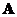and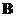are said to be equal if the size of the two matrices are equal and all of the corresponding elements are equal, that is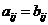for each row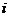and column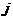.

Example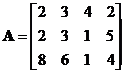,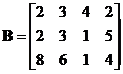Since all corresponding elements are equal, we say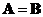Example: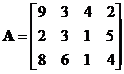,All corresponding elements seem equal but the first element is not equal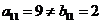we say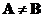Very simple interactive program below is designed to answer question whether the two input matrices are equal or not. Try with your own input matrix . Try also different decimal precision of your input.

compare to